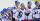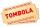# Bureau of Labor

Bureau of Labor is a state institution that provides mike and the rest for their so-called clients. The mission of the Bureau of Labor is spend taxpayer money to provide relaxation and benefits to those who do not want to work. Popularly speaking sense the work of the Bureau of Labor is to create the largest number of unemployed. This helps him massively Labour Code, trade unions, minimum wage and other regulations that were not here.

Back to mathematics. Probability that Bureau of Labor "finds" unemployed job is 1.7%. Probability that a job seeker finds job as active person is 93%.

Calculate how many times the probability of finding a job more his own efforts than through Bureau of Labor.

Result

x =  54.7

#### Solution:

$x = \dfrac{ 93} { 1.7} = 54.7$

Leave us a comment of this math problem and its solution (i.e. if it is still somewhat unclear...):Be the first to comment!#### Following knowledge from mathematics are needed to solve this word math problem:

Need help calculate sum, simplify or multiply fractions? Try our fraction calculator. Our percentage calculator will help you quickly calculate various typical tasks with percentages. Would you like to compute count of combinations?

## Next similar math problems:

1. Boys and girlsThere are eight boys and nine girls in the class. There were six children on the trip from this class. What is the probability that left a) only boys b) just two boys
2. STRESSED wordEach letter in STRESSED is printed on identical cards, one letter per card and assembled in random order. Calculate the probability that the cards spell DESSERTS when assembled.
3. WordWhat is the probability that a random word composed of chars E, Y, G, E, R, O, M, T will be the GEOMETRY?
4. Hockey playersAfter we cycle five hockey players sit down. What is the probability that the two best scorers of this crew will sit next to each other?
5. Dices throwsWhat is the probability that the two throws of the dice: a) Six falls even once b) Six will fall at least once
6. Sum or productWhat is the probability that two dice fall will have the sum 7 or product 12?
7. CardsFrom a set of 32 cards we randomly pull out three cards. What is the probability that it will be seven king and ace?
8. Boys and girlsThere are 11 boys and 18 girls in the classroom. Three pupils will answer. What is the probability that two boys will be among them?
9. SalamiHow many ways can we choose 5 pcs of salami if we have 6 types of salami for 10 pieces and one type for 4 pieces?
10. ClassroomOf the 26 pupils in the classroom, 12 boys and 14 girls, four representatives are picked to the odds of being: a) all the girls b) three girls and one boy c) there will be at least two boys
11. Three-digit numbersHow many three-digit numbers are from the numbers 0 2 4 6 8 (with/without repetition)?
12. Combinations of sweatersI have 4 sweaters two are white, 1 red and 1 green. How many ways can this done?
13. Word MATEMATIKAHow many words can be created from the word MATEMATIKA by changing the order of the letters, regardless of whether or not the words are meaningful?
14. Families 2There are 729 families having 6 children each. The probability of a girl is 1/3 and the probability of a boy is 2/3. Find the the number of families having 2 girls and 4 boys.
15. RaffleThere are 200 draws in the raffle, but only 20 of them win. What is the probability of at least 4 winnings for a group of people who have bought 5 tickets together?
16. Three workplacesHow many ways can we divide nine workers into three workplaces if they need four workers in the first workplace, 3 in the second workplace and 2 in the third?
17. Two groupsThe group of 10 girls should be divided into two groups with at least 4 girls in each group. How many ways can this be done?